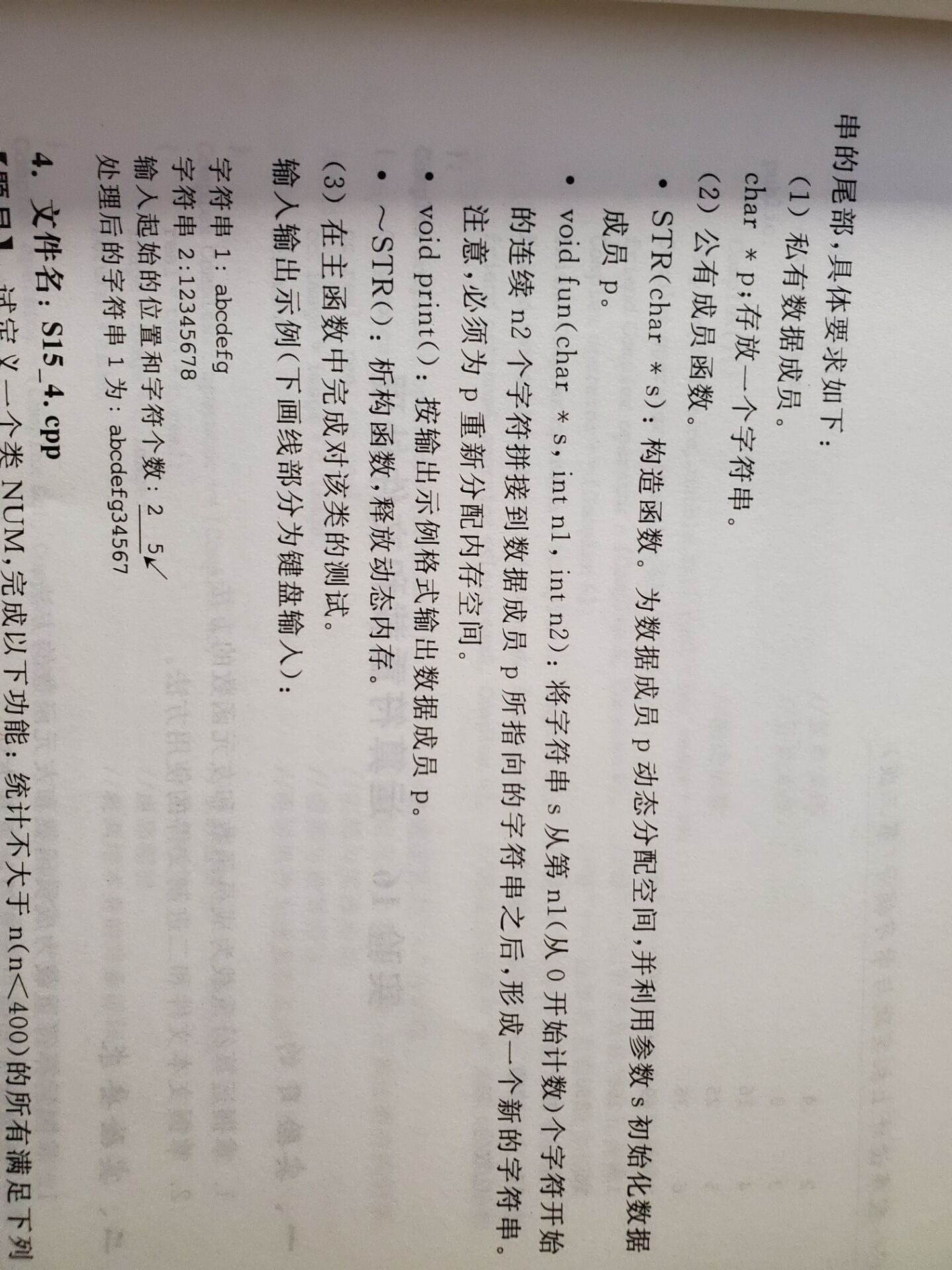2021-06-03 14:09

# C++编程题求大佬解答• 点赞
• 写回答
• 关注问题
• 收藏
• 邀请回答

#### 1条回答默认 最新

•qfl_sdu 2021-06-03 15:01
已采纳

#include <iostream>

#include <string>

using namespace std;

class STR

{

private:

char* p;

public:

STR(char* s){

int len= strlen(s);

p = new char[len+1];

p[len] = '\0';

memcpy(p,s,len);

}

~STR(){delete[d] p;}

void fun(char* s,int n1, int n2){

char* tmp = new char[strlen(p)+1+n2];

memcpy(tmp,p,strlen(p));

strcpy(tmp+strlen(p),s+n1,n2 );

tmp[strlen(p) +n2 ] = '\0';

delete[] p;

p = tmp;

tmp = 0;

}

void print(){

cout << p << endl;

}

}

int main(){

int n1,n2;

char s1[] = "abcdefg";

char s2[] = "12345678";

cout << "字符串1:" << s1 << endl;

cout << "字符串2:" <<s2 << endl;

cout <<"请输出起始位置和字符数:";

cin >> n1 >> n2;

STR tt(s1);

tt.fun(s2,n1,n2);

tt.print();

return 0;

}

点赞 打赏 评论